Many modern autonomous systems (such as autonomous cars or robots1) rely on “reward functions”, which encode the robot’s desired behavior; in other words, reward functions effectively tell a robot, in any given situation, which action is good and which is bad.

Today, in practice, reward functions are designed by hand via an iterative process of trial-and-error. Such an approach is not only time-consuming but, more concerningly, very prone to failures. For example, consider a simple autonomous driving task:It may seem reasonable to choose a reward function that incentivizes the robot to maintain a high speed on the road; however, when an autonomous car is provided with said reward function, it instead does the following: (Credit: Mat Kelcey)The approach of hand-designing reward functions scales poorly with the complexity of the system: as robots and their tasks become more complex, it becomes increasingly more difficult for a system designer to design a reward function that encodes good behavior in every possible setting the robot may encounter2. Thus, if we want to deploy robots in the infinitely-complex real world, we’re going to need a better approach.

One such approach is to learn the reward function directly from humans. This can be done in a variety of ways: by having the human demonstrate the task to the robot, by querying the human directly to learn their preferences, by having humans physically provide feedback to the robot while it is operating, etc. In reward learning, and more generally in problems that involve learning from humans, we typically pick one such mode of feedback and use data of that form when learning. In this work however, we investigate, in the specific context of reward learning, how we can _combine multiple modes of human feedback to learn from humans more effectively. _But first, we discuss some current state-of-the-art approaches to reward learning.

Inverse Reinforcement Learning. Inverse Reinforcement Learning (IRL)3 is one popular approach for learning reward functions. IRL leverages the idea that while a human may not be able to specify a reward function for the task, they can instead show the robot how to perform the task. In IRL, a human provides demonstrations of the robot’s desired behavior on the specified task; the algorithm then infers a reward function from these demonstrations. For example, in autonomous driving, the human may provide demonstrations of them driving a car themselves; or in robotics, the expert human may provide demonstrations by teleoperating the robot using a controller.

IRL can work remarkably well when the demonstrations are of high-quality. However, this is rarely the case in robotics. Consider, for example, the simple task below where the robot needs to smoothly move its arm to the black pad while avoiding the obstacle (as in the marked blue line):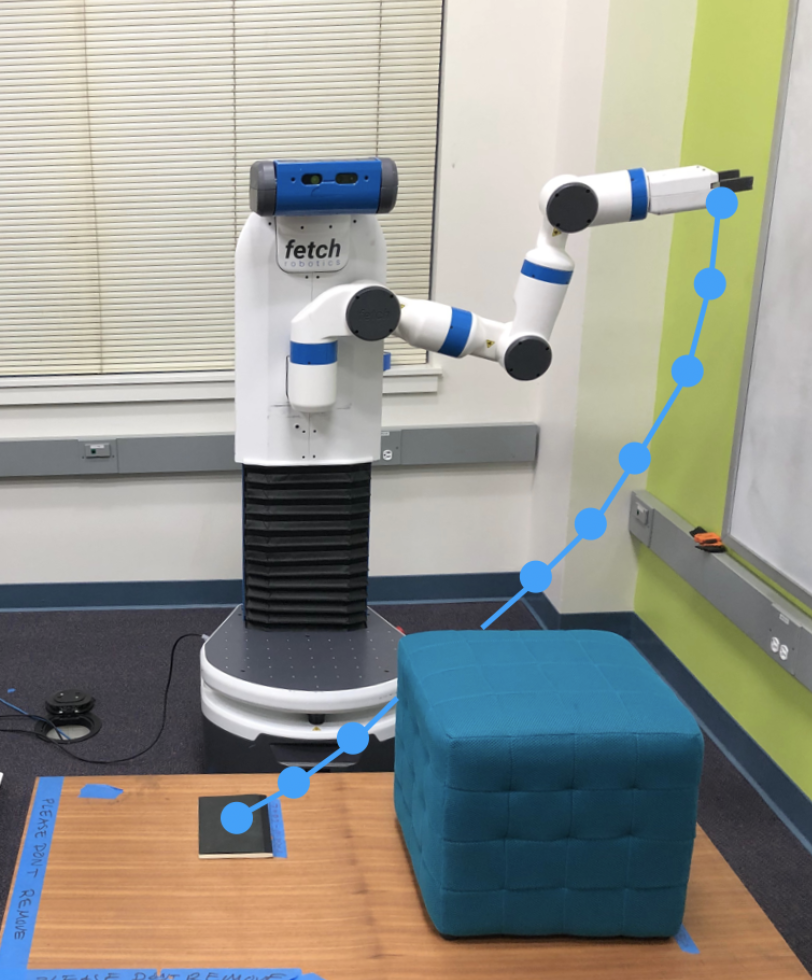We tasked several users with providing demonstrations on this task using a simple joystick controller. One such user’s demonstration is shown below: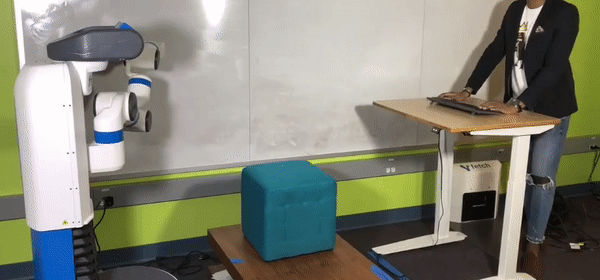The above user had extensive prior experience with controlling robots, but despite this, we can see that (1) the user doesn’t manage to fully get the robot’s arm to the goal and that (2) his demonstration is extremely choppy. Other users remarked about the system:

1. “I had a hard time controlling the robot.”
2. “[It] would be nice if the controller for the [robot] was easier to use”

It is difficult to control complex robots with many joints using intuitive joystick controllers since the robot has many more degrees-of-freedom (i.e., joints) than the joystick does. While more complex controllers may resolve this issue, people still struggle to provide high-quality demonstrations with such controllers since they are unintuitive to use. So, if people cannot provide effective good demonstrations, how else can we learn the reward function?

Preference-Based Learning. In such situations where users cannot provide informative demonstrations, we can instead learn about the true reward function by using structured queries to elicit that information directly from the user. One such method for doing this, known as preference-based learning, queries the user for her preference between two examples of the robot attempting the task. Each of the user’s responses encode some information about the true reward function. Therefore, by repeatedly querying the user we can infer the true reward function. For example, in the robotics task discussed above, the user may be presented with the following two trajectories in a preference query: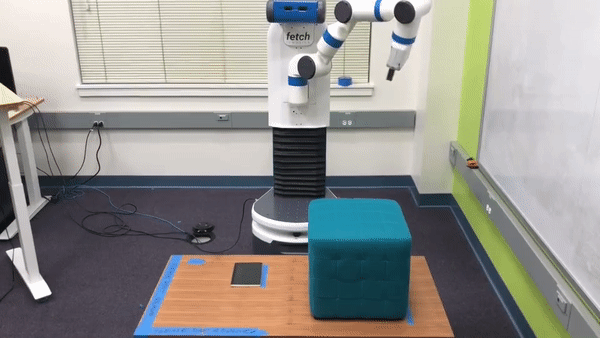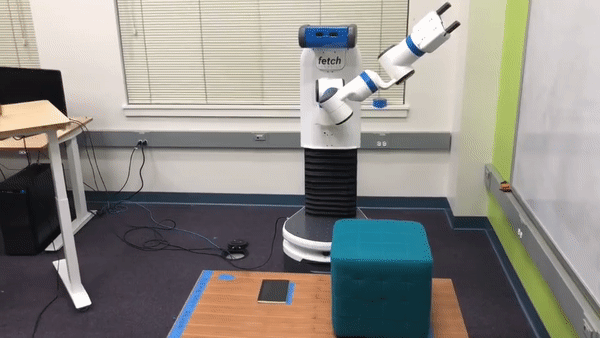While neither trajectory perfectly accomplishes the task, the trajectory on top gets much closer to the goal and so we would expect the human to pick that one. This process of generating a pair of trajectories and querying the human for her preference between them is repeated many times until the algorithm can accurately infer a reward function for the robot.

It is much easier for a human to accurately respond to a preference query, i.e., choose between two trajectories, than to provide a full-length demonstration. Thus, we expect the human input in preference-based learning to be of very high quality; as a result, we expect the learned reward function to accurately reflect the human’s desired behavior for the robot.

However, preference-based learning methods face several challenges of their own. Most notably, these methods tend to be very data-inefficient. Each preference query gives us little information about the true reward function, especially when compared to a full-length demonstration. Since the space of all possible reward functions is very large and complex, it often takes many, many preference queries – and therefore, a lot of human time – to find the true reward function with preference-based learning.

Our key insight is that preference queries are comparatively more accurate but less informative than demonstrations. In our work, we leverage this insight to derive a new framework for reward learning, DemPref. DemPref, as the name suggests, utilizes both demonstrations and preferences to learn an accurate reward function efficiently by amplifying each of their respective benefits and dampening their respective drawbacks.

## DemPref

In DemPref, we learn the reward function in two stages. In the first stage, the human provides demonstrations, which are used to construct a probabilistic distribution over reward functions. This can be done in several ways, but we choose to do so using Bayesian IRL4, where we learn a distribution by performing a Bayesian update over all possible reward functions ($R$ here denotes a given reward function, and $\xi_i$ denotes the $i$th human demonstration):

$P(R\mid\xi_1,\ldots,\xi_n) \propto \exp\left(\sum_{i=1}^n R(\xi_i))\right)$

This distribution can be thought of as a prior over reward functions, which upweights those reward functions more likely to be the true reward function while downweighting those less likely. Since the demonstrations are imperfect, this prior over reward functions will be imperfect as well; however, this prior still contains valuable information about the true reward function and allows us to effectively shrink the space of possible reward functions.

Hence, in the second stage of DemPref, we can use a preference-based learning algorithm with this prior to find the true reward function much more quickly than if were starting with no prior, since we now only have to search over a much smaller space of possible reward functions. Many different preference-based learning algorithms can be used here, and we choose to use an active preference-based learning algorithm based on the maximum volume removal method5; this algorithm attempts to generate at each step, the query that is most informative about the true reward function and therefore generally requires fewer queries than other methods.

By specifically avoiding using preference queries at the initial stages and demonstrations at the latter stages, our method diminishes the impact of their relatively uninformative and inaccurate nature respectively. In other words, we leverage (1) the information-rich nature of demonstrations to speed up the process of learning the reward function and (2) the accurate nature of preference queries to ensure that the reward function we learn is accurate.

Our simulated experimental results show that using DemPref significantly reduces the number of queries required to learn the true reward function, when compared to the same preference-based learning algorithm that doesn’t use any demonstrations.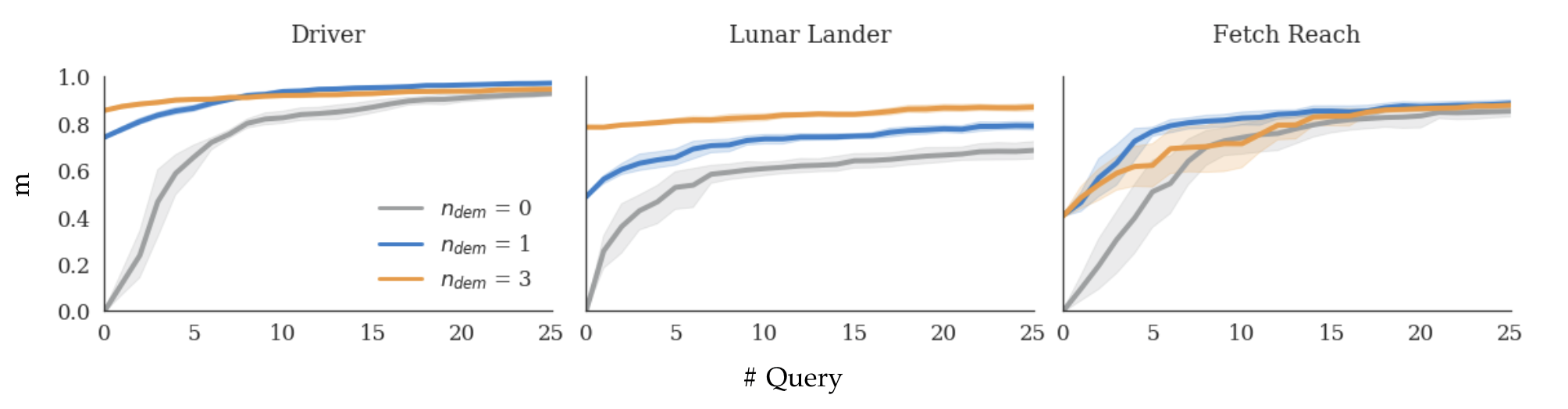Evaluation of DemPref in simulation. Here, $n_{dem}$ is the number of demonstrations used in DemPref; $n=0$ corresponds to standard preference-based learning. $m$ measures how close the learned reward function is to the true reward function; $m =1$ implies that the learned reward function is exactly the true reward function.

### Dealing with non-convexity

One challenge with the active preference-based learning algorithm we use is that it generates the queries at each step by approximately solving a non-convex optimization problem: these problems are especially difficult to solve because they have many possible solutions which “look optimal” to optimization algorithms, but which are actually sub-optimal. Hence, for complex problems, the algorithm can end up generating a query in which neither option yields much information about the human’s true reward function. See, for example, the uninformative query below, which the algorithm generated for the robotics task from above: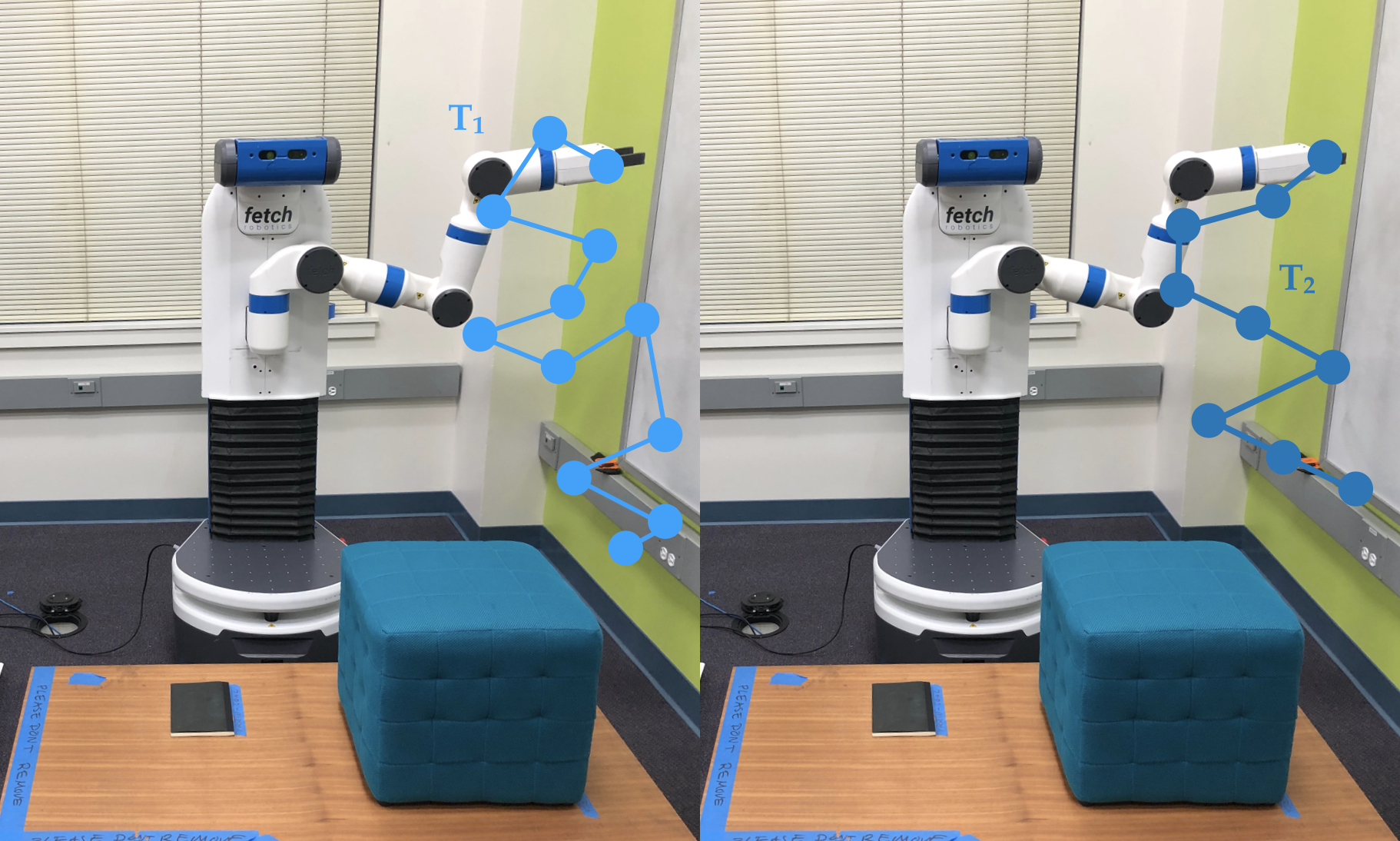Since neither trajectory reflects the desired behavior of the robot, it is very difficult for a human to choose between the two trajectories in a manner that is consistent with their internal reward function. Hence, the human’s answers to such queries can mislead the algorithm and significantly slow down the process of learning the true reward function. This is a common problem with such preference-based learning methods and was first identified by Bıyık and Sadigh (2018)6.

In our work, we derive a new preference-based learning algorithm to be used in conjunction with DemPref, that can alleviate this issue. In the standard DemPref framework, the demonstration is only useful in learning a prior to generate preference queries with. However, we instead retain the demonstration during the second phase, and use them to ground the preference queries: at each step, we let the human choose between the two generated trajectories or the demonstration she initially provided. If the human chooses one of the generated trajectories, that trajectory will take the place of the demonstration in the next set of preference queries. This ensures that there is always at least one trajectory in the query that is reasonably reflective of the human’s true reward function:We can think of this preference-based learning method as iteratively finding better trajectories, starting from the initial demonstration; hence, we refer to this preference-based learning method as Iterated Correction (IC).

In IC, when the human has to respond to a preference query, she now has to pick between three trajectories, instead of two. However, by only asking the human to pick her most preferred of the three trajectories, we are discarding valuable information since the human’s preference between the remaining two trajectories also contains information about the true reward function. Hence, in our algorithm, we instead allow the human to rank all three trajectories, instead of simply specifying her preference between them. \

Our simulation experiments show that DemPref with IC significantly reduces the number of queries required to learn the true reward function, over standard DemPref: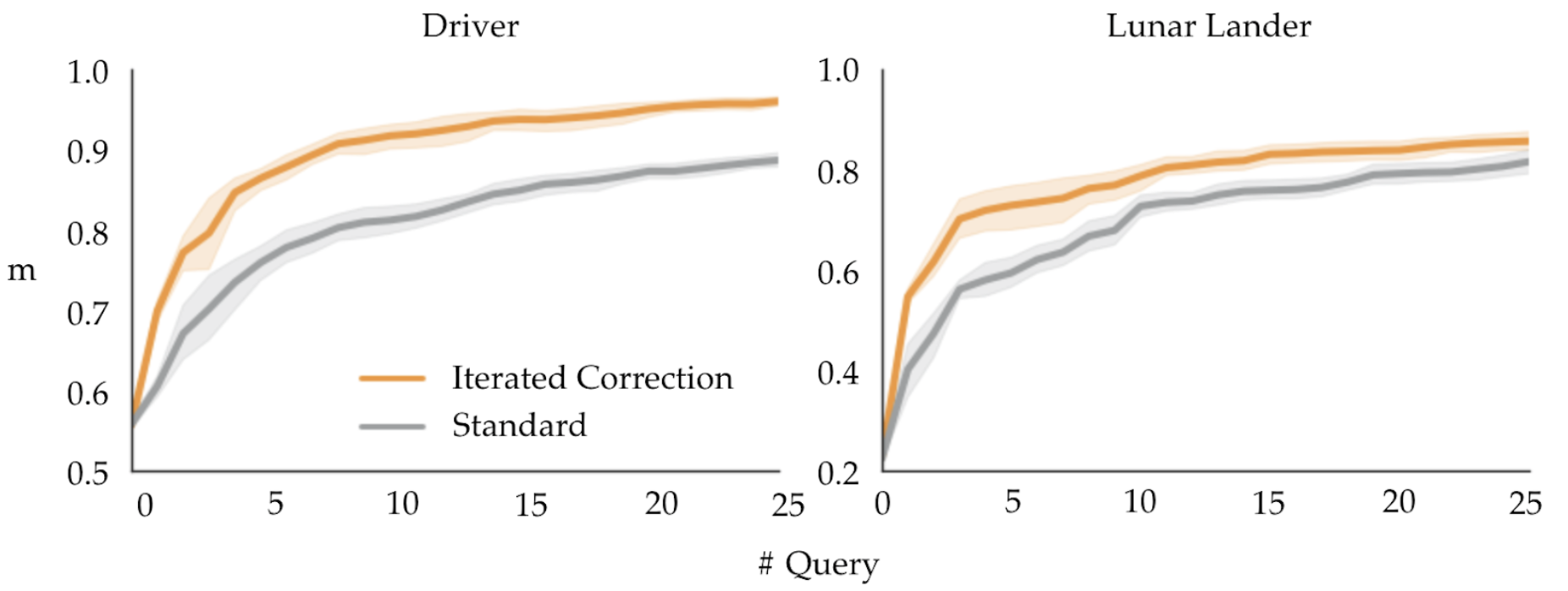The following diagram summarizes our full algorithm: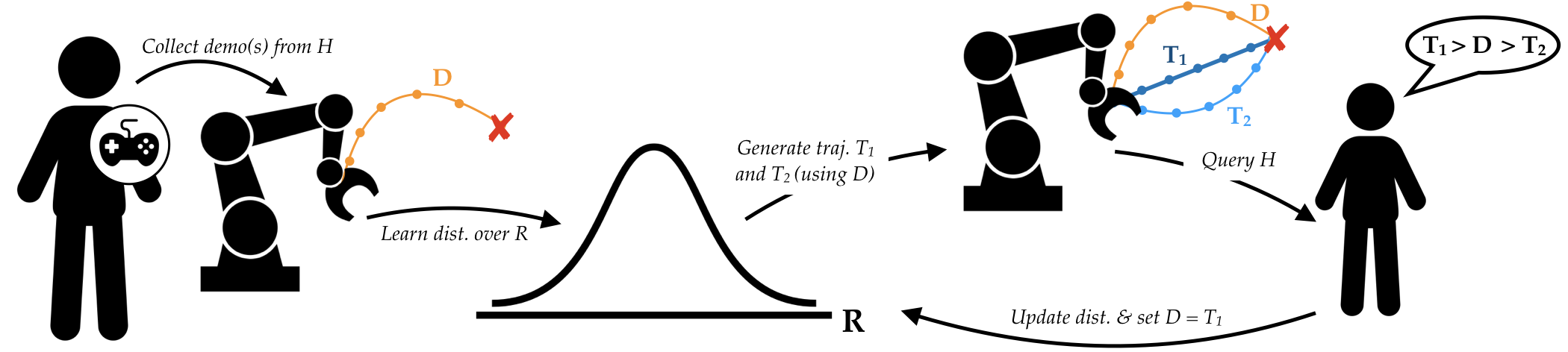User study. Thus far we have shown that DemPref is indeed more sample efficient than prior preference based learning algorithms, but have yet to demonstrate that it allows for more accurate learning over prior IRL algorithms. A common application for IRL is enabling humans to teach real, physical robots to accomplish specific tasks7. Hence, to investigate whether DemPref was more effective than IRL at learning reward functions from real humans for this application, we ran a user study with 15 participants. Each of the users were tasked at training the robot on the same robotics task discussed above using both DemPref and IRL. The learned reward function was then used to train the robot on the same task, but in a slightly different domain. (Below, the training environment is shown on the left, while the testing environment is shown on the right. Desired trajectories are shown in blue in both diagrams.)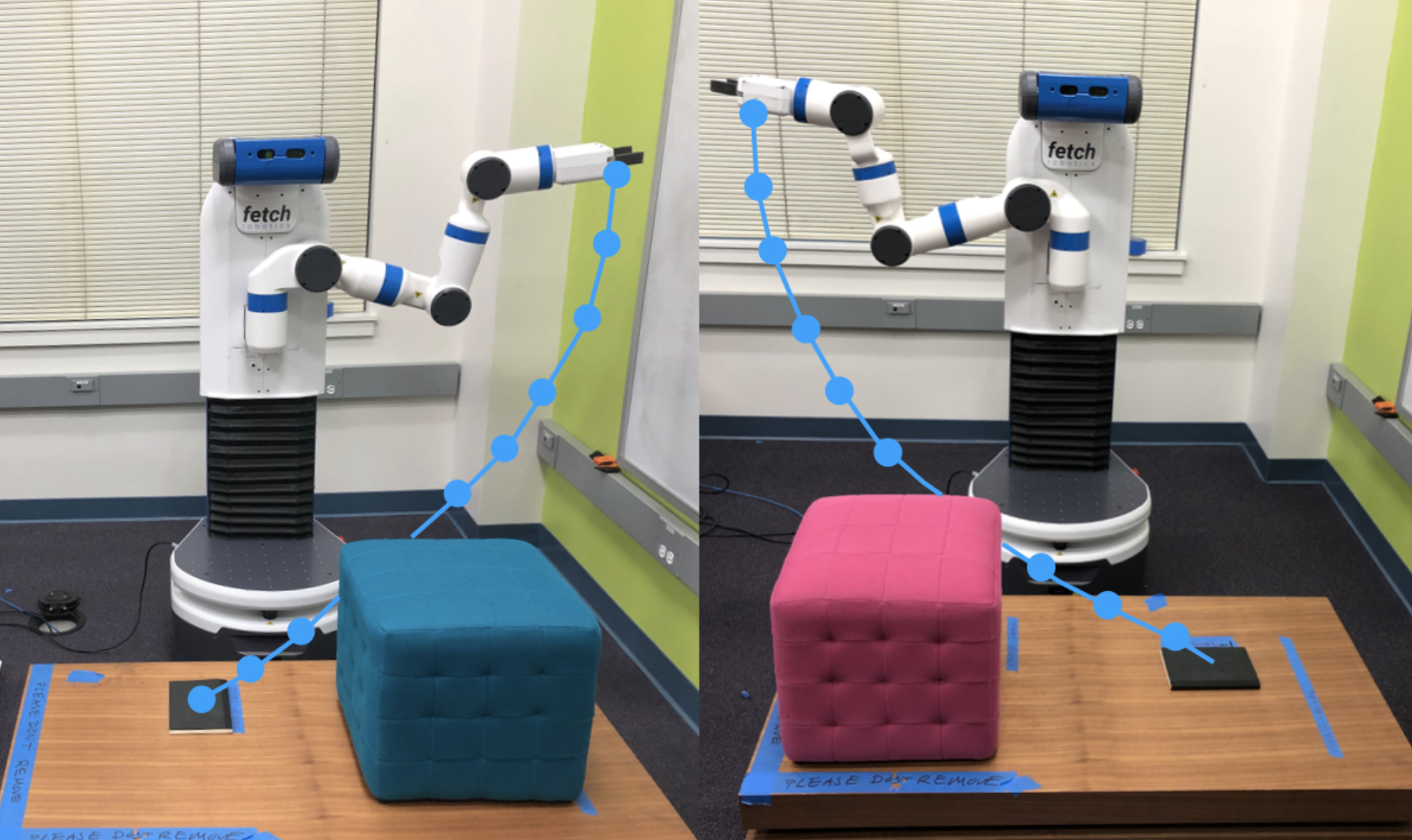Below, we show an example (filmed during a user study) of the behavior of the IRL robot (top) and the DemPref robot (bottom):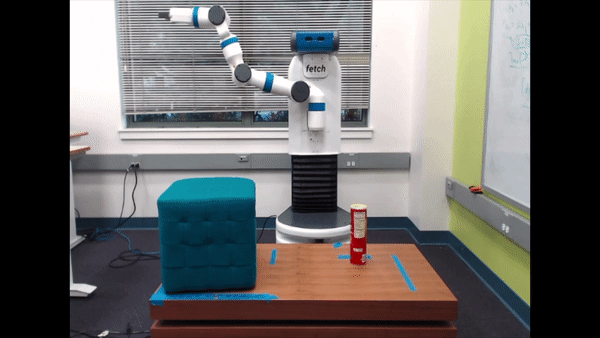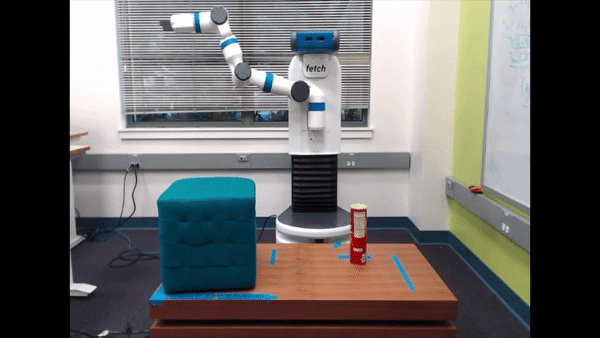Here, the IRL robot tries so hard to avoid the obstacle that it doesn’t even bother with trying to reach the goal; this behavior was induced by the low-quality demonstrations received from the user. In contrast, the DemPref robot did not suffer from these issues, since the feedback received from preference queries were of a high-quality.

This was not an isolated incident; many users encountered similar issues with the IRL robot. When the users were asked to rate the behavior of the robots trained with DemPref and the robot trained with IRL on the new task, the users overwhelmingly rated the DemPref robot as being better at the task than the IRL robot. Additionally, when asked about their preferences between the two methods for training the robot, the users also overwhelmingly preferred the DemPref robot over the IRL robot.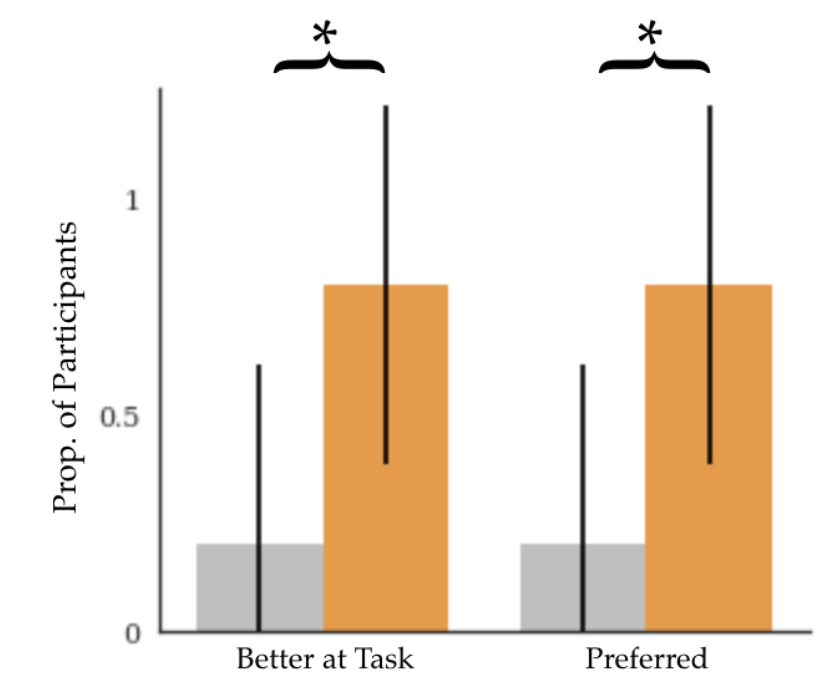User study preferences among the IRL robot (grey) and DemPref robot (orange). The asterisk denotes statistically significant differences.

## Future work

It is interesting to note that despite the challenges that users faced with controlling the robot, they did not rate the DemPref system as being “easier” to use than the IRL system.

This was largely due to the time-taken to generate each query in the preference-based learning stage. Recall that the preference-based learning algorithm generated queries by solving a non-convex (hard!) optimization problem. This optimization is fairly time-consuming; for example, in our task, it took roughly ~45 seconds to generate each query. Since each query is generated according to the human’s previous answers, the queries cannot be precomputed or parallelized either. Several users specifically referred to the time it took to generate each query as negatively impacting their experience with the DemPref system: “I wish it was faster to generate the preference [queries]”, “In overall human time the [IRL] system… actually took less time”, “The [DemPref system] will be even better if time cost is less.”

Additionally, one user expressed difficulty in evaluating the preference queries themselves, commenting that “It was tricky to understand/infer what the preferences were [asking]. Would be nice to communicate that somehow to the user (e.g. which [trajectory] avoids collision better)!” These are valid critiques of the framework, and indeed, we are currently investigating approaches to (a) generate preferences more quickly via distributed optimization and (b) generate more interpretable preferences as directions for future work.

## Conclusion

In this work, we showed that we can learn reward functions more efficiently and accurately by leveraging multiple modes of human feedback in such a way that amplifies their respective strengths while dampening their respective weaknesses. While our work was grounded in the context of reward learning, we are optimistic about the potential that this fundamental idea – of leveraging data from multiple modes of human feedback in a structured manner – holds more broadly for the field of learning from humans. We believe that, applied correctly, this idea can improve the process of learning from humans greatly, in a large number of domains.

This blog post is based on the following paper that will appear at RSS 2019:

Learning Reward Functions by Integrating Human Demonstrations and Preferences. Malayandi Palan*, Nicholas C. Landolfi*, Gleb Shevchuk, Dorsa Sadigh. RSS, 2019. PDF

* Denotes equal contribution.

This project was partially supported by grants from Toyota Research Institute and Future of Life Institute. We are grateful for the helpful feedback provided by Michelle Lee and Andrey Kurenkov.

1. We henceforth use the term robots to refer generically to an autonomous system.

2. See here for an extensive list of well-known examples where hand-designed reward functions fail (sometimes catastrophically).

3. Often referred to as Learning from Demonstrations (LfD), and closely related to Imitation Learning.

4. Ramachandran, Deepak, and Eyal Amir. “Bayesian Inverse Reinforcement Learning.” IJCAI, 2007.

5. Sadigh, Dorsa, Anca D. Dragan, Shankar Sastry, and Sanjit A. Seshia. “Active preference-based learning of reward functions.” RSS, 2017.

6. Bıyık, Erdem, and Dorsa Sadigh. “Batch Active Preference-Based Learning of Reward Functions.” CORL, 2018.

7. Usually, it would not be practical to run a preference-based learning algorithm on a real, high degree-of-freedom robot such as a manipulator, as the algorithm would be too slow; however, using DemPref, we are able to learn reward functions on real robots due to the efficiency gains from additionally using demonstrations.